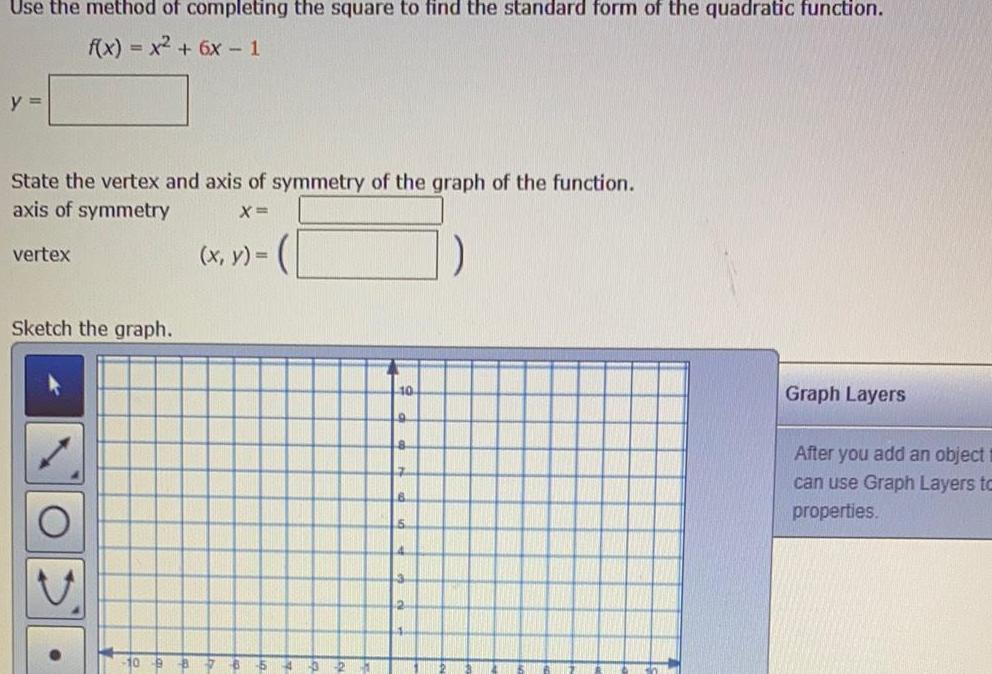Math
Parabola
Use the method of completing the square to find the standard form of the quadratic function f x x 6x 1 y State the vertex and axis of symmetry of the graph of the function axis of symmetry X vertex Sketch the graph O x y 10 9 8 Graph Layers After you add an object can use Graph Layers to properties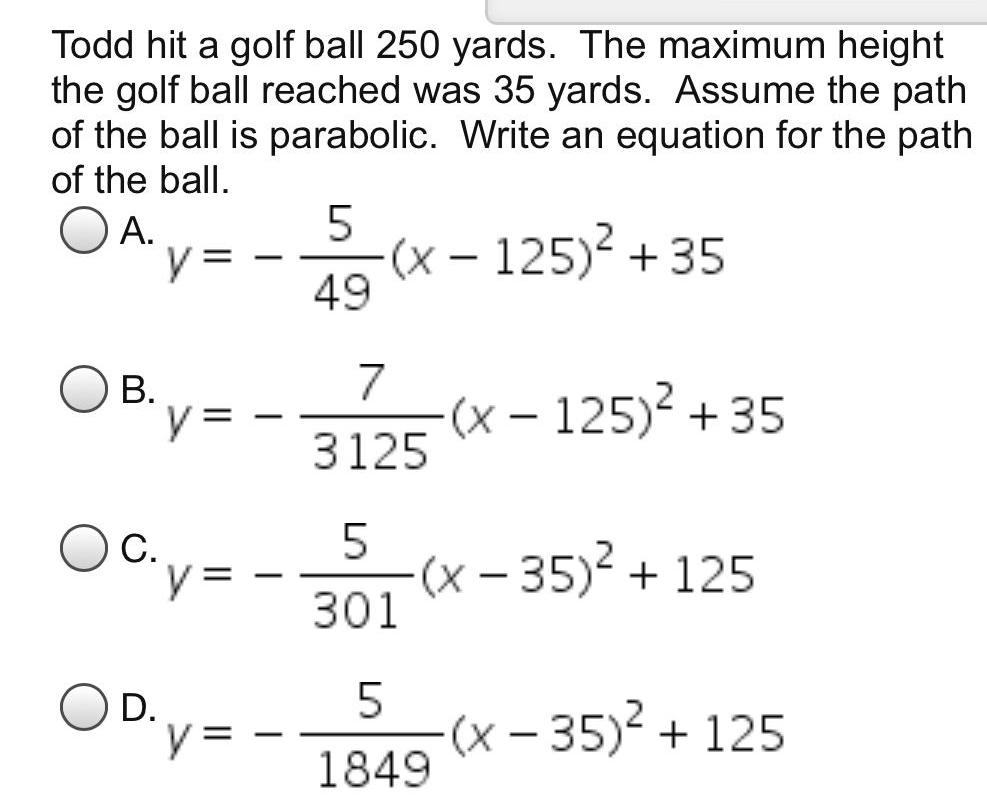Math
Parabola
Todd hit a golf ball 250 yards The maximum height the golf ball reached was 35 yards Assume the path of the ball is parabolic Write an equation for the path of the ball OA B C D y y y y 5 49 x 125 35 7 3125 x 125 35 5 301 x 35 125 5 1849 x 35 125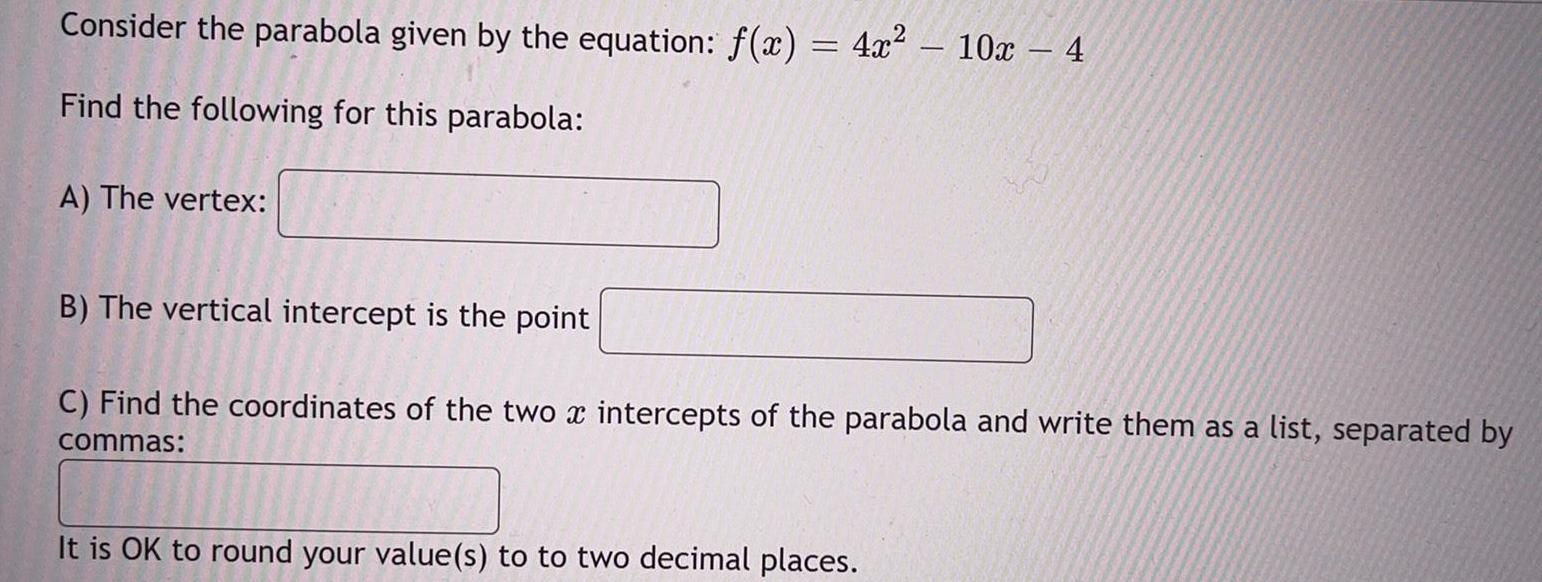Math
Parabola
Consider the parabola given by the equation: f(x) = 4x² – 10x-4 Find the following for this parabola: A) The vertex: B) The vertical intercept is the point C) Find the coordinates of the two x intercepts of the parabola and write them as a list, separated by commas: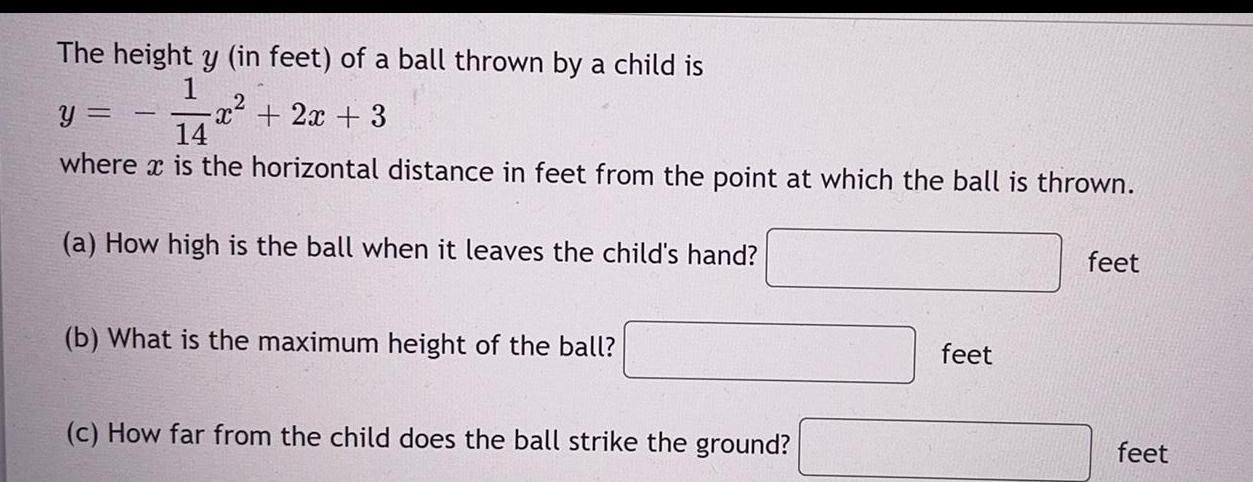Math
Parabola
The height y (in feet) of a ball thrown by a child is y=-1/14 x² + 2x + 3 where is the horizontal distance in feet from the point at which the ball is thrown. (a) How high is the ball when it leaves the child's hand? feet (b) What is the maximum height of the ball? feet (c) How far from the child does the ball strike the ground? feet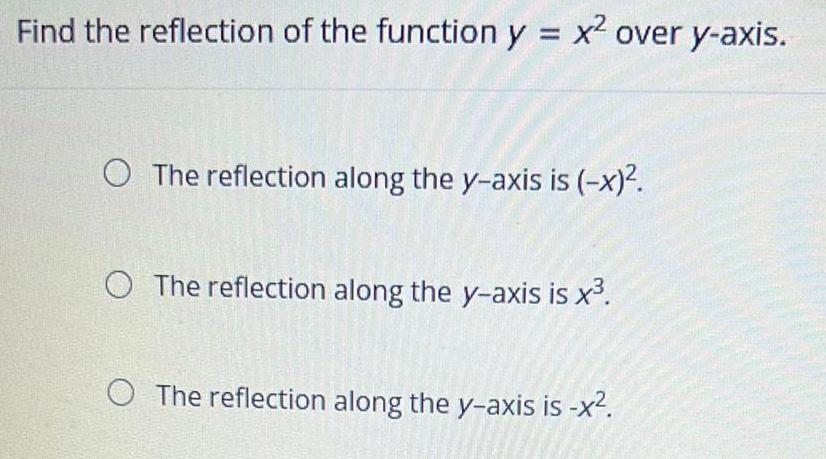Math
Parabola
Find the reflection of the function y = x² over y-axis. The reflection along the y-axis is (-x)². The reflection along the y-axis is x³. The reflection along the y-axis is -x².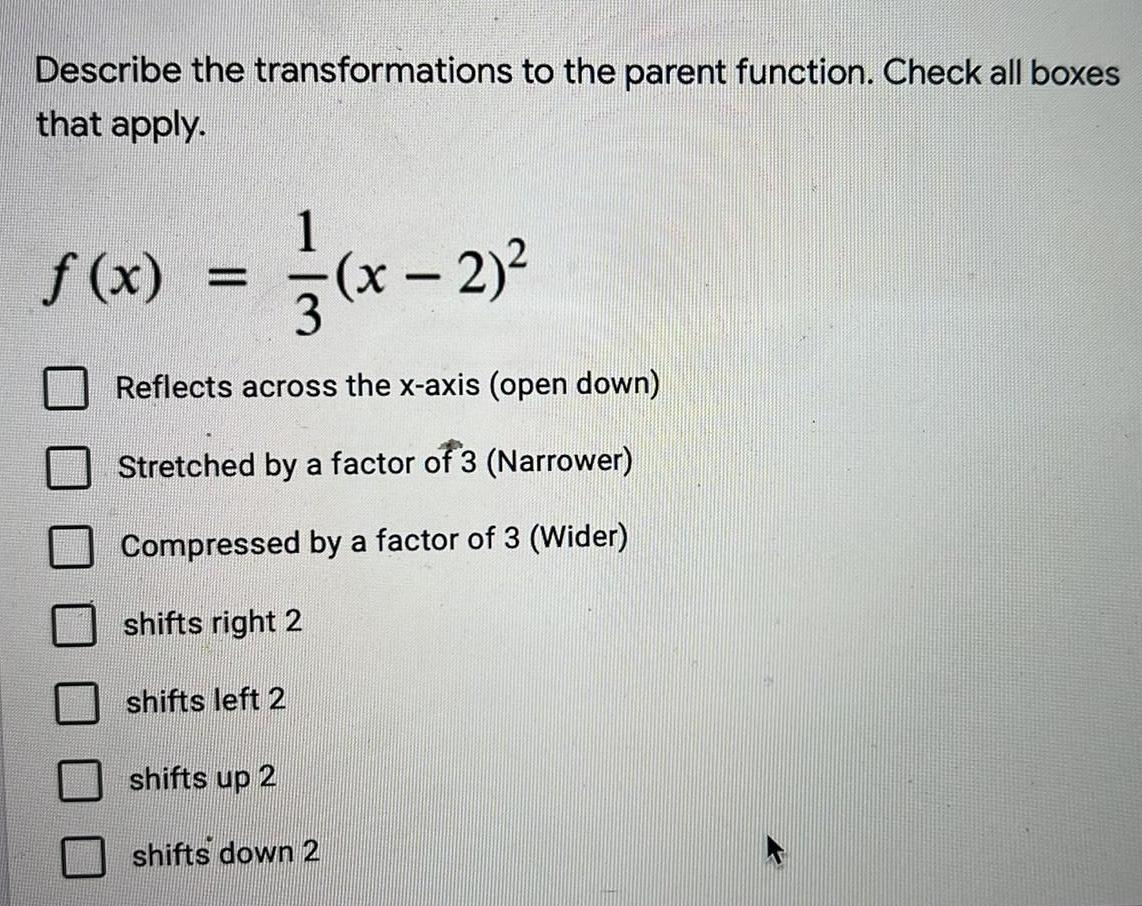Math
Parabola
Describe the transformations to the parent function. Check all boxes that apply. f(x)=1/3(x - 2)² Reflects across the x-axis (open down) Stretched by a factor of 3 (Narrower) Compressed by a factor of 3 (Wider) shifts right 2 shifts left 2 shifts up 2 shifts down 2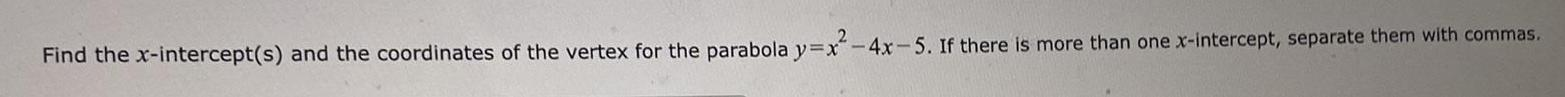Math
Parabola
Find the x-intercept(s) and the coordinates of the vertex for the parabola y=x²-4x-5. If there is more than one x-intercept, separate them with commas.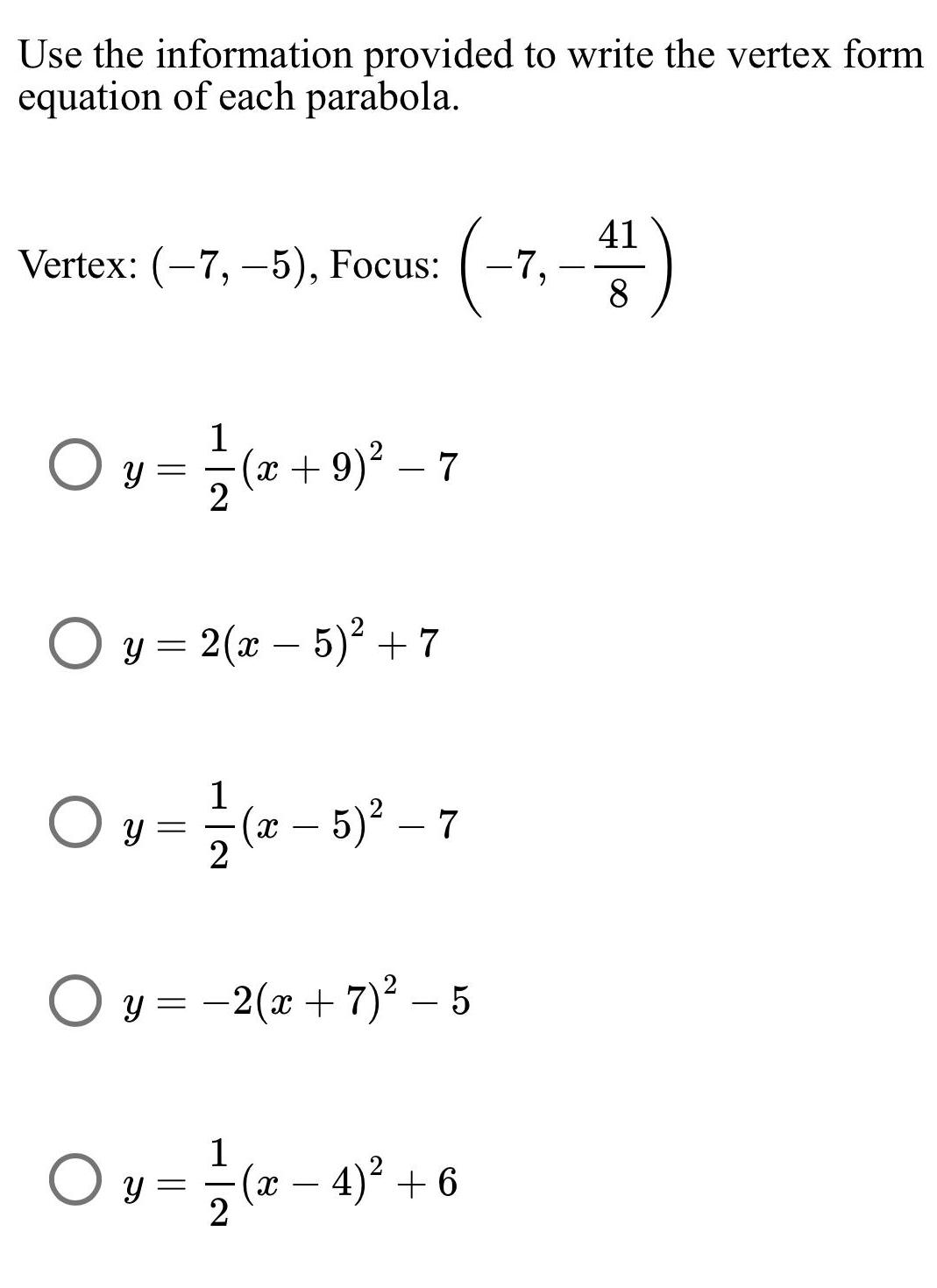Math
Parabola
Use the information provided to write the vertex form equation of each parabola. Vertex: (-7,-5), Focus: (-7,-41/8) y = (x +9) ² - 7 y = 2(x - 5)² +7 y- 1/2(x-5)²-7 y = -2(x+7)² - 5 y = 1/2( x − 4 )² + 6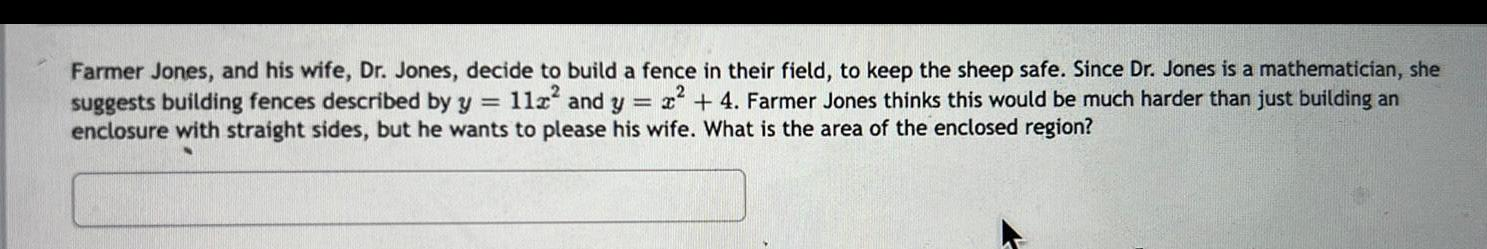Math
Parabola
Farmer Jones, and his wife, Dr. Jones, decide to build a fence in their field, to keep the sheep safe. Since Dr. Jones is a mathematician, she suggests building fences described by y = 112² and y = x² + 4. Farmer Jones thinks this would be much harder than just building an enclosure with straight sides, but he wants to please his wife. What is the area of the enclosed region?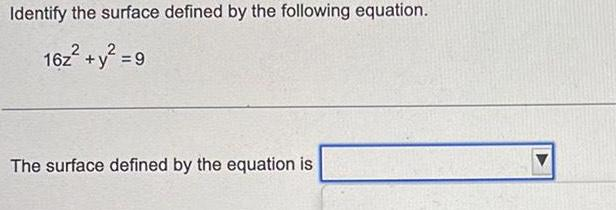Math
Parabola
Identify the surface defined by the following equation. 16z² + y² = 9 The surface defined by the equation is _____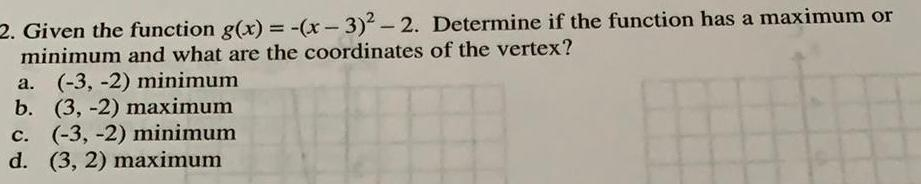Math
Parabola
Given the function g(x) = -(x-3)² - 2. Determine if the function has a maximum or minimum and what are the coordinates of the vertex? a. (-3, -2) minimum b. (3,-2) maximum c. (-3, -2) minimum d. (3, 2) maximum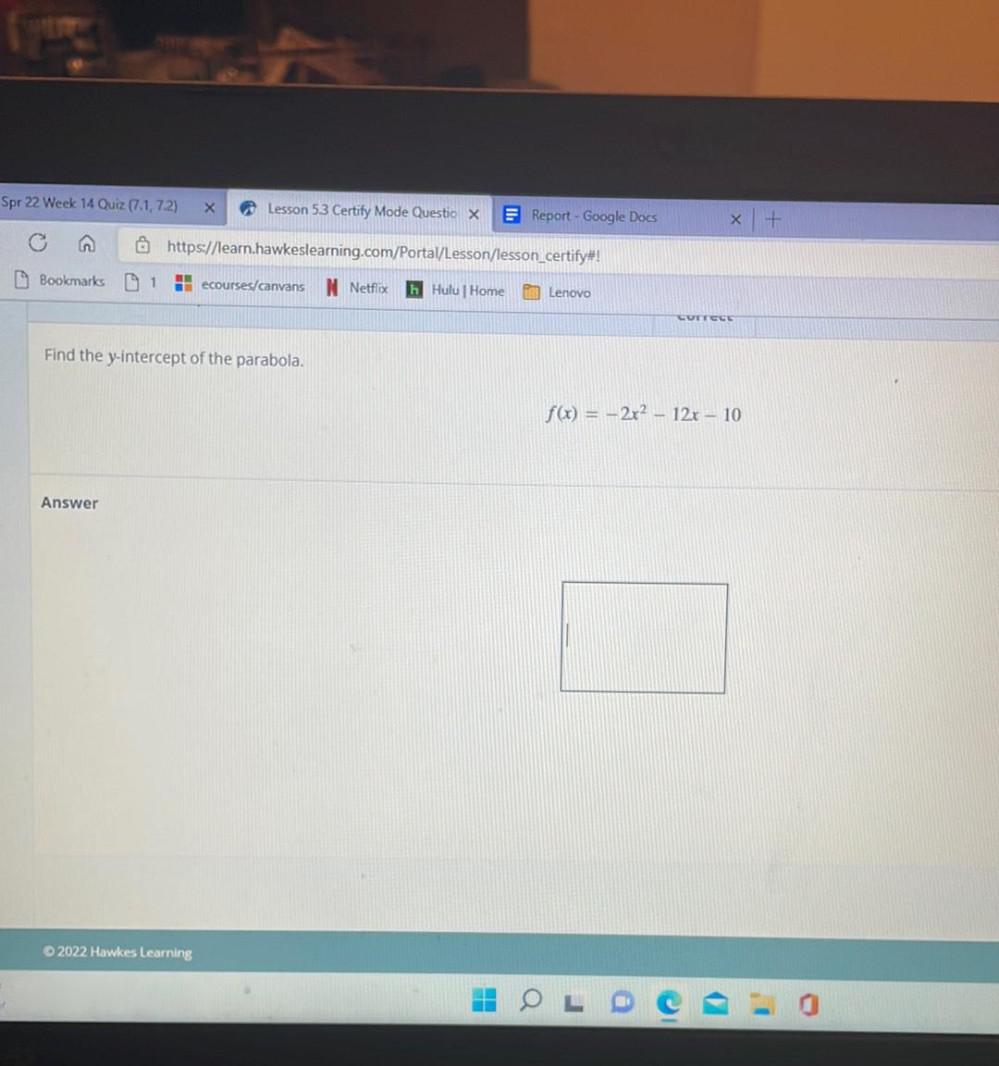Math
Parabola
Find the y-intercept of the parabola. f(x) = -2x^2-12x-10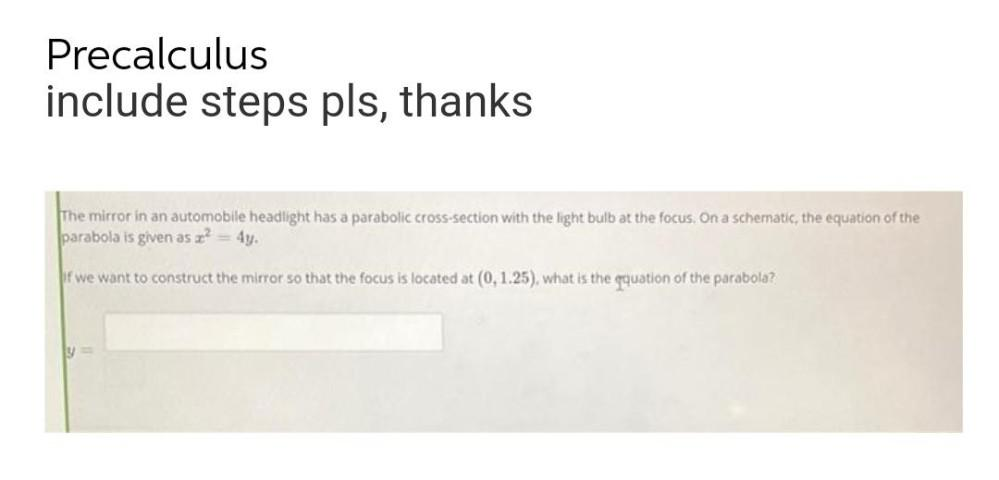Math
Parabola
The mirror in an automobile headlight has a parabolic cross-section with the light bulb at the focus. On a schematic, the equation of the parabola is given as x^2= 4y. if we want to construct the mirror so that the focus is located at (0,1.25), what is the equation of the parabola?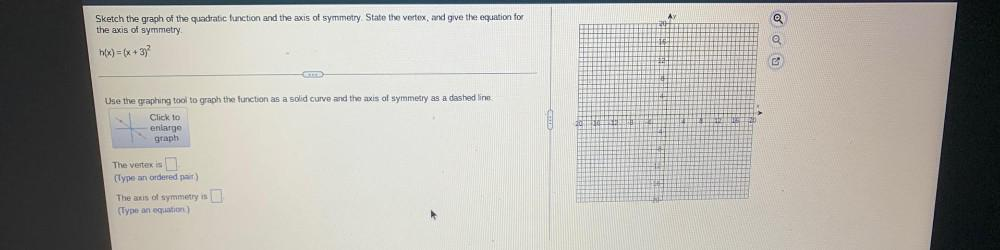Math
Parabola
Sketch the graph of the quadratic function and the axis of symmetry. State the vertex, and give the equation for the axis of symmetry. h(x) = (x + 3)^2 Use the graphing tool to graph the function as a solid curve and the axis of symmetry as a dashed line. Click to enlarge graph. The vertex is ____. (Type an ordered pair.) The axis of symmetry is _______. (Type an equation.)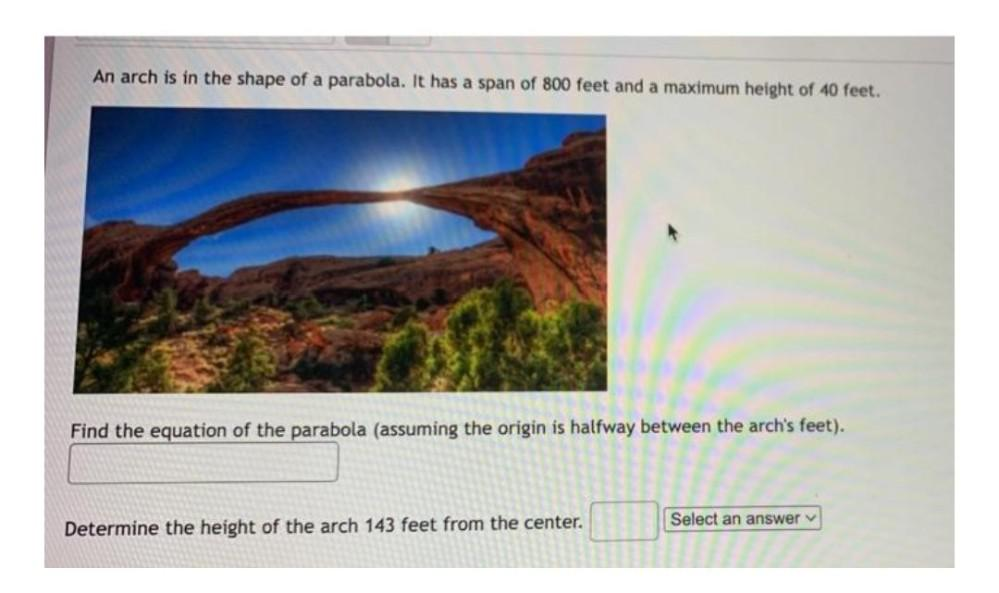Math
Parabola
An arch is in the shape of a parabola. It has a span of 800 feet and a maximum height of 40 feet. Find the equation of the parabola (assuming the origin is halfway between the arch's feet).Determine the height of the arch 143 feet from the center.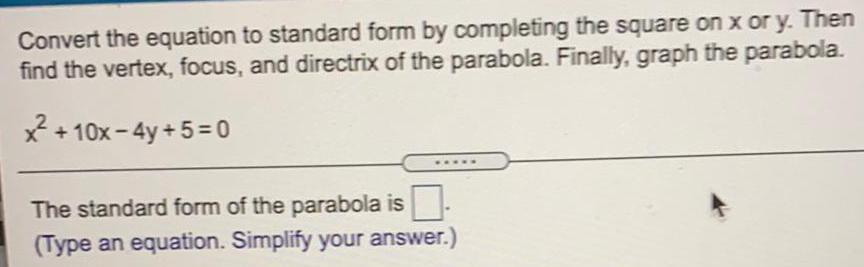Math
Parabola
Convert the equation to standard form by completing the square on x or y. Then find the vertex, focus, and directrix of the parabola. Finally, graph the parabola. x² +10x-4y+5=0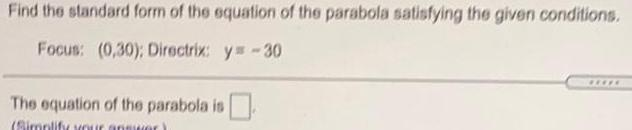Math
Parabola
Find the standard form of the equation of the parabola satisfying the given conditions. Focus: (0,30); Directrix: y=-30 The equation of the parabola is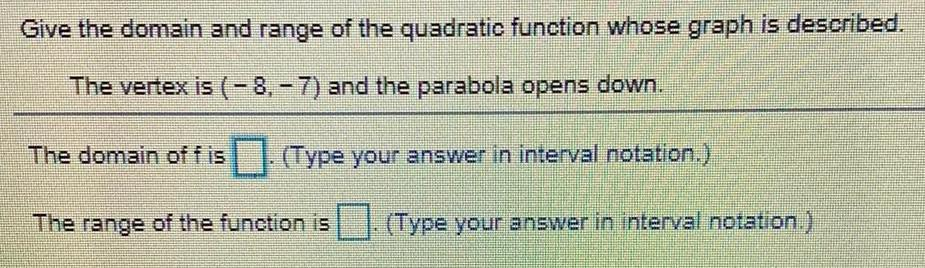Math
Parabola
Give the domain and range of the quadratic function whose graph is described. The vertex is (-8, -7) and the parabola opens down. The domain off is. (Type your answer in interval notation.) The range of the function is (Type your answer in interval notation.)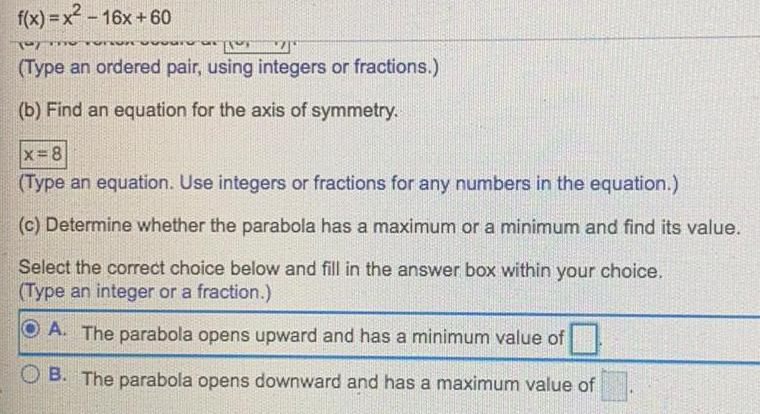Math
Parabola
f(x)=x²-16x +60 (Type an ordered pair, using integers or fractions.) (b) Find an equation for the axis of symmetry. x=8 (Type an equation. Use integers or fractions for any numbers in the equation.) (c) Determine whether the parabola has a maximum or a minimum and find its value. Select the correct choice below and fill in the answer box within your choice. (Type an integer or a fraction.) A. The parabola opens upward and has a minimum value of B. The parabola opens downward and has a maximum value of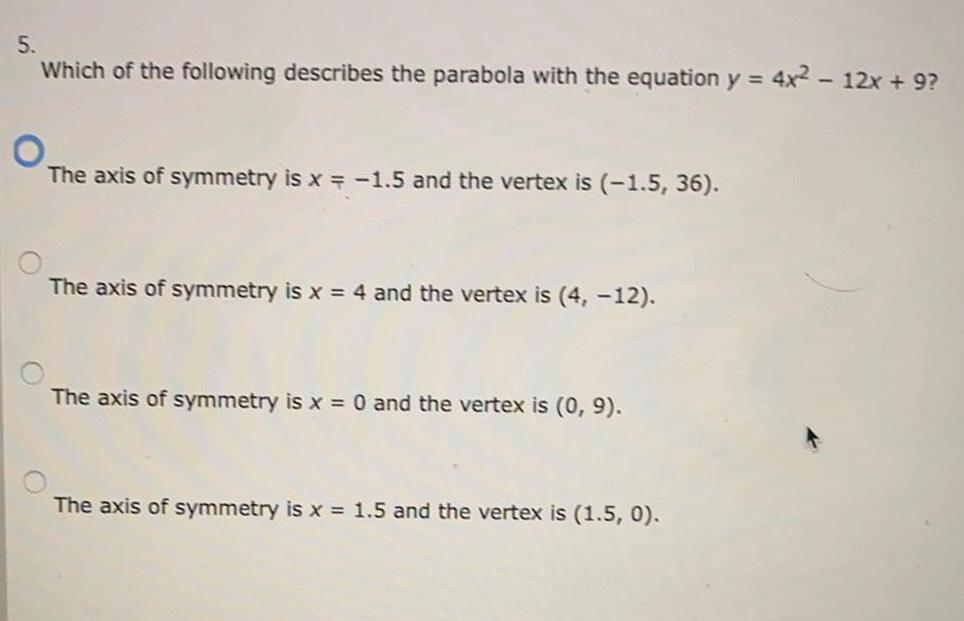Math
Parabola
Which of the following describes the parabola with the equation y = 4x² - 12x + 9? The axis of symmetry is x = -1.5 and the vertex is (-1.5, 36). The axis of symmetry is x = 4 and the vertex is (4, -12). The axis of symmetry is x = 0 and the vertex is (0, 9). The axis of symmetry is x = 1.5 and the vertex is (1.5, 0).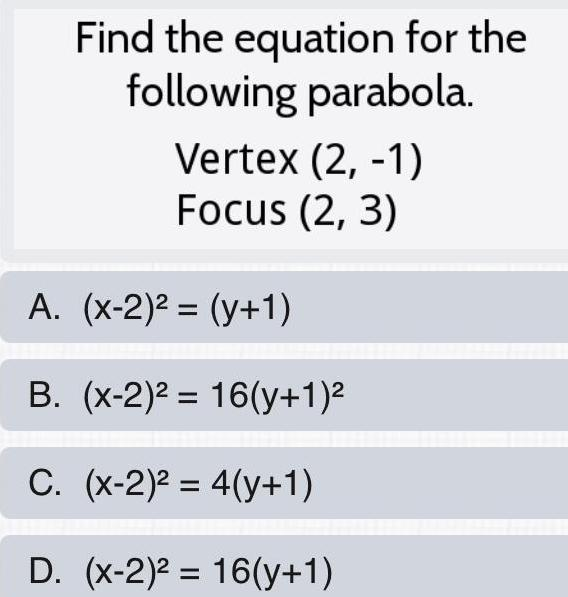Math
Parabola
Find the equation for the following parabola. Vertex (2, -1) Focus (2, 3) A. (x-2)² = (y+1) B. (x-2)² =16(y+1)² C. (x-2)² = 4(y+1) D. (x-2)² 16(y+1)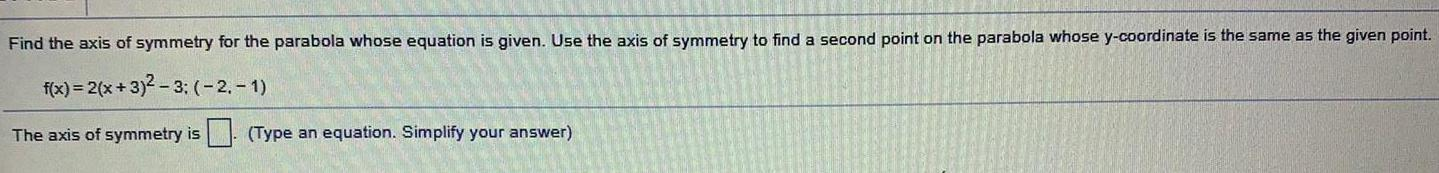Math
Parabola
Find the axis of symmetry for the parabola whose equation is given. Use the axis of symmetry to find a second point on the parabola whose y-coordinate is the same as the given point. f(x) = 2(x+3)²-3; (-2,-1) The axis of symmetry is (Type an equation. Simplify your answer)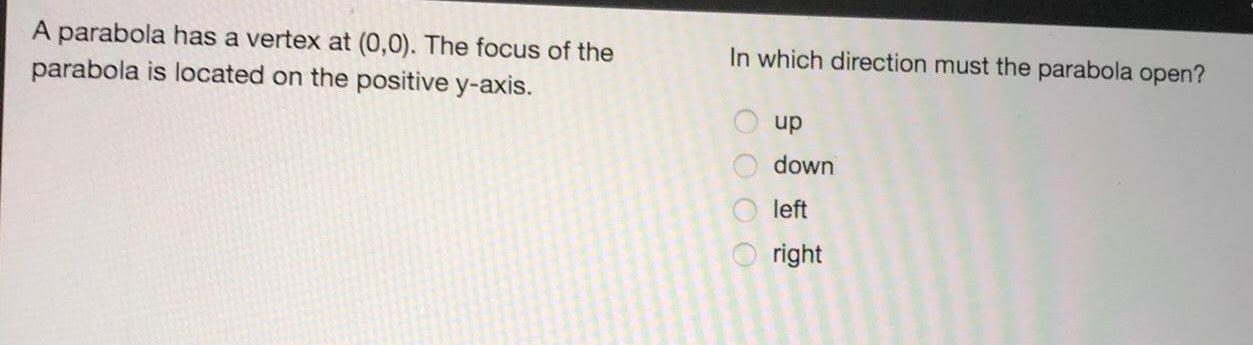Math
Parabola
A parabola has a vertex at (0,0). The focus of the parabola is located on the positive y-axis. In which direction must the parabola open? up down left right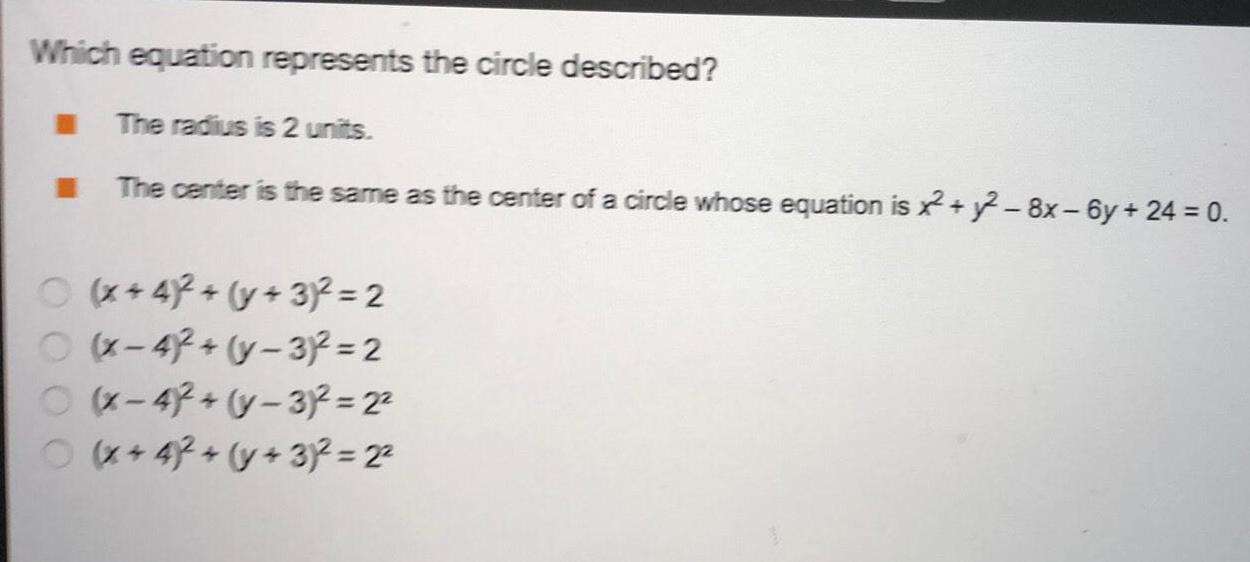Math
Parabola
Which equation represents the circle described? The radius is 2 units. The center is the same as the center of a circle whose equation is ²2+2-8x-6y + 24 = 0. (x+4)2 + (y + 3)² = 2 (x-4)2+(y-3)²=2 (x-4)2+(y-3)² =2² (x+42 + (y + 3)² = 2²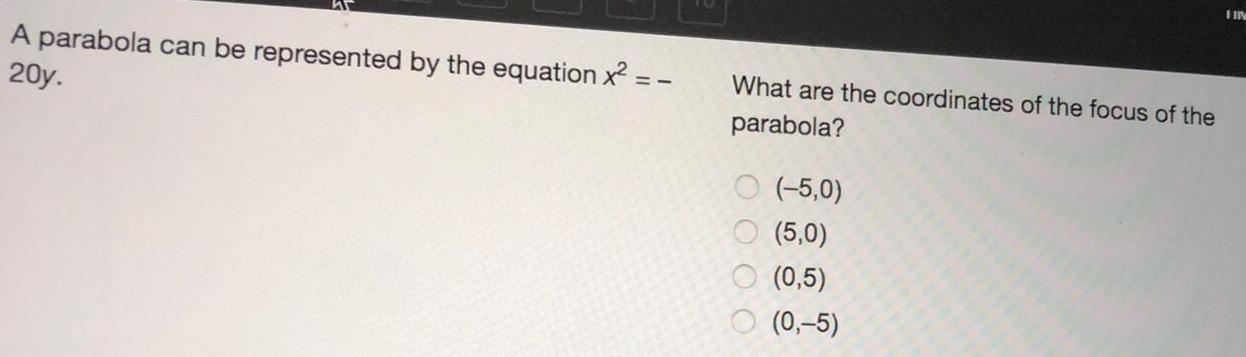Math
Parabola
A parabola can be represented by the equation x² = - 20y. What are the coordinates of the focus of the parabola? (-5,0) (5,0) (0,5) (0,-5)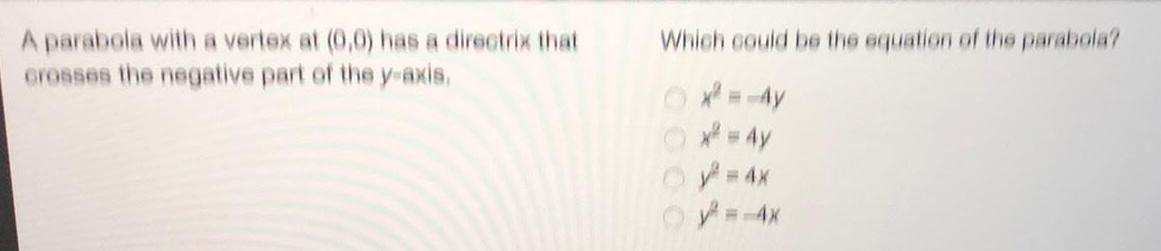Math
Parabola
A parabola with a vertex at (0,0) has a directrix that crosses the negative part of the y-axis. Which could be the equation of the parabola? x^2 = -4y x² = 4y y² = 4x y=-4x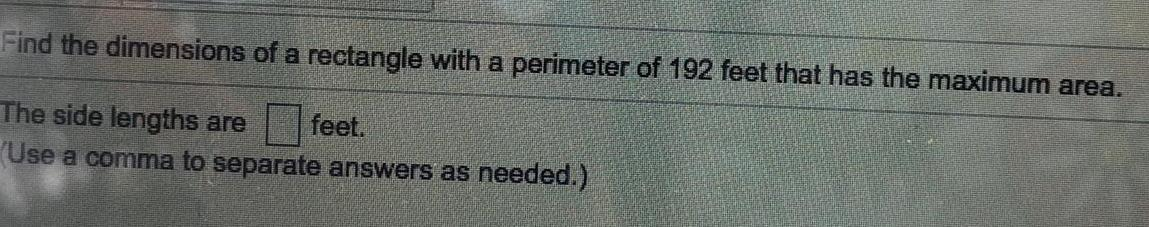Math
Parabola
Find the dimensions of a rectangle with a perimeter of 192 feet that has the maximum area. The side lengths are feet. (Use a comma to separate answers as needed.)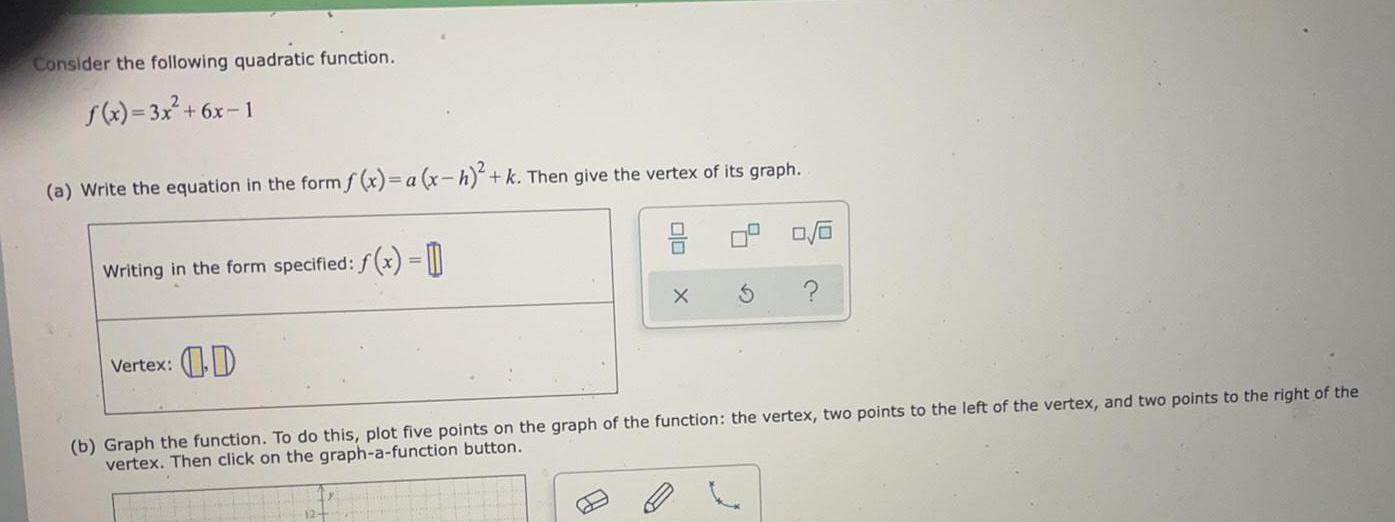Math
Parabola
Consider the following quadratic function. f(x)= 3x² +6x-1 (a) Write the equation in the form f(x)= a (x-h)²+ k. Then give the vertex of its graph. Writing in the form specified: f(x) = Vertex: (b) Graph the function. To do this, plot five points on the graph of the function: the vertex, two points to the left of the vertex, and two points to the right of the vertex. Then click on the graph-a-function button.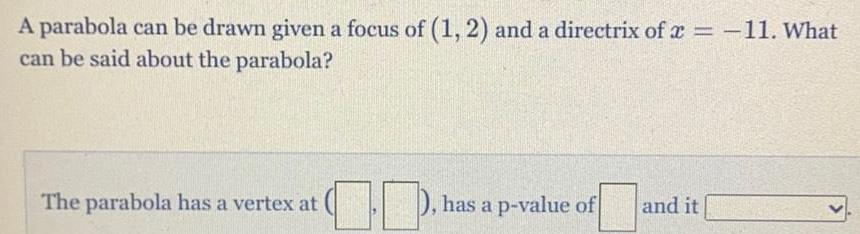Math
Parabola
A parabola can be drawn given a focus of (1, 2) and a directrix of x = -11. What can be said about the parabola? The parabola has a vertex at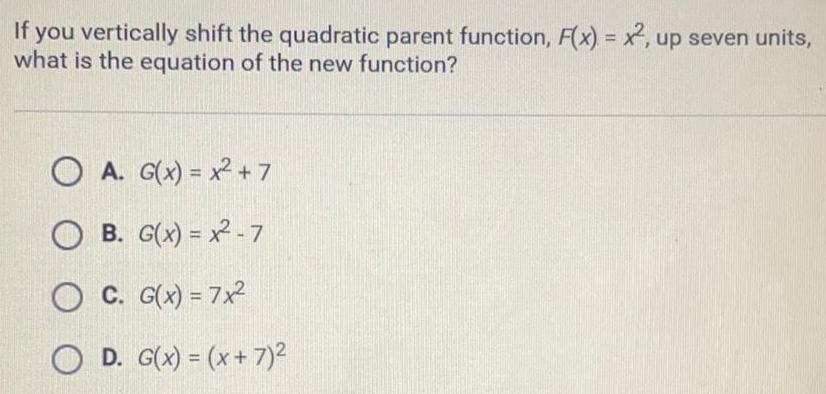Math
Parabola
If you vertically shift the quadratic parent function, F(x) = x2, up seven units, what is the equation of the new function? A. G(x) = x² +7 B. G(x)=x2-7 C. G(x) = 7x² D. G(x) = (x + 7)²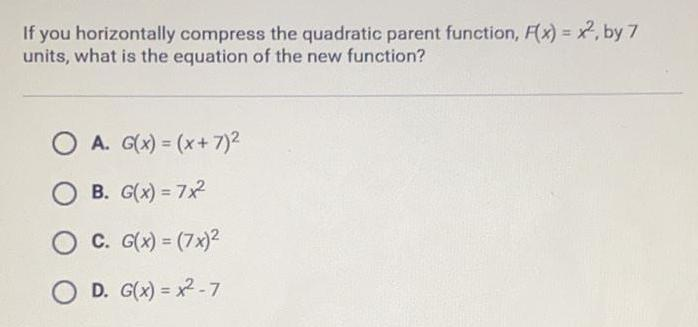Math
Parabola
If you horizontally compress the quadratic parent function, F(x)=x2, by 7 units, what is the equation of the new function? A. G(x) = (x+7)² B. G(x) = 7x² C. G(x) = (7x)² D. G(x)=x²-7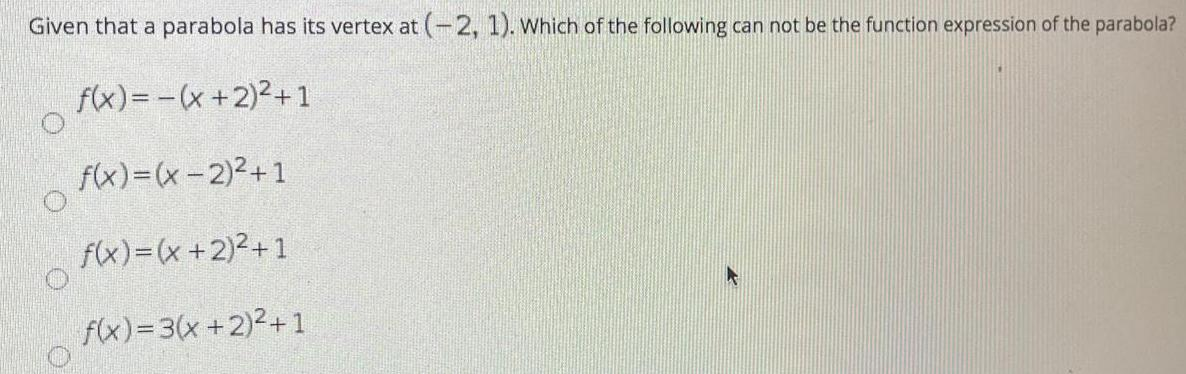Math
Parabola
Given that a parabola has its vertex at (-2, 1). Which of the following can not be the function expression of the parabola? f(x) = -(x + 2)²+1 f(x)=(x-2)²+1 f(x)=(x + 2)²+1 f(x)=3(x+2)²+1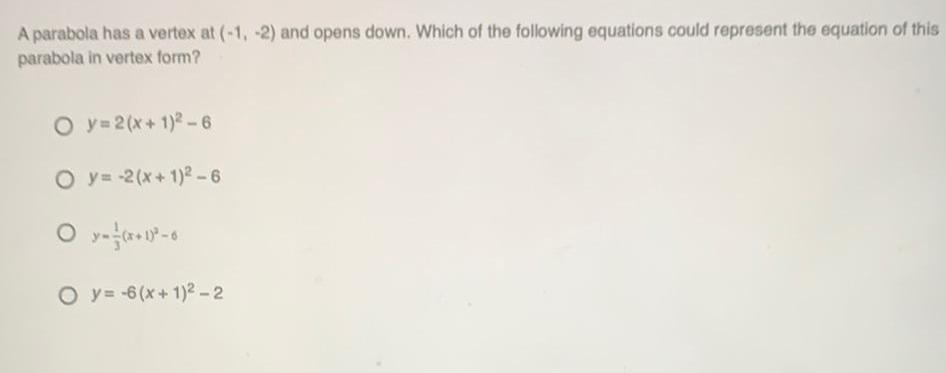Math
Parabola
A parabola has a vertex at (-1, -2) and opens down. Which of the following equations could represent the equation of this parabola in vertex form? y=2(x+1)2-6 y=-2(x+1)²-6 y= 1/3 (x+1)²-6 y=-6(x+1)²-2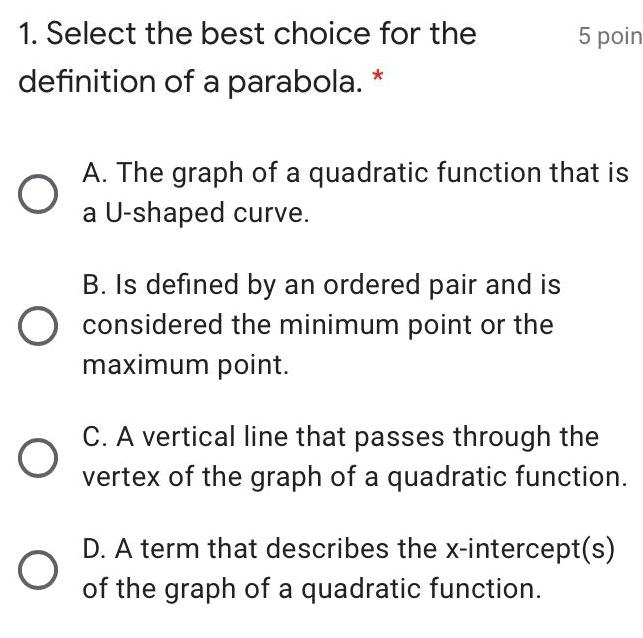Math
Parabola
1. Select the best choice for the definition of a parabola. * A. The graph of a quadratic function that is a U-shaped curve. B. Is defined by an ordered pair and is considered the minimum point or the maximum point. C. A vertical line that passes through the vertex of the graph of a quadratic function. D. A term that describes the x-intercept(s) of the graph of a quadratic function.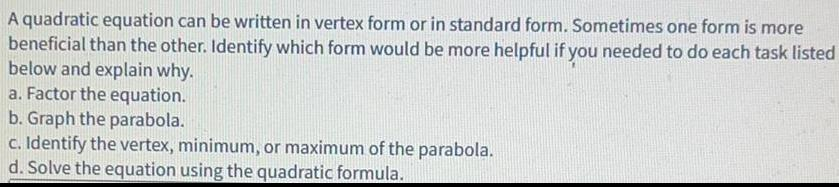Math
Parabola
A quadratic equation can be written in vertex form or in standard form. Sometimes one form is more beneficial than the other. Identify which form would be more helpful if you needed to do each task listed below and explain why. a. Factor the equation. b. Graph the parabola. c. Identify the vertex, minimum, or maximum of the parabola. d. Solve the equation using the quadratic formula.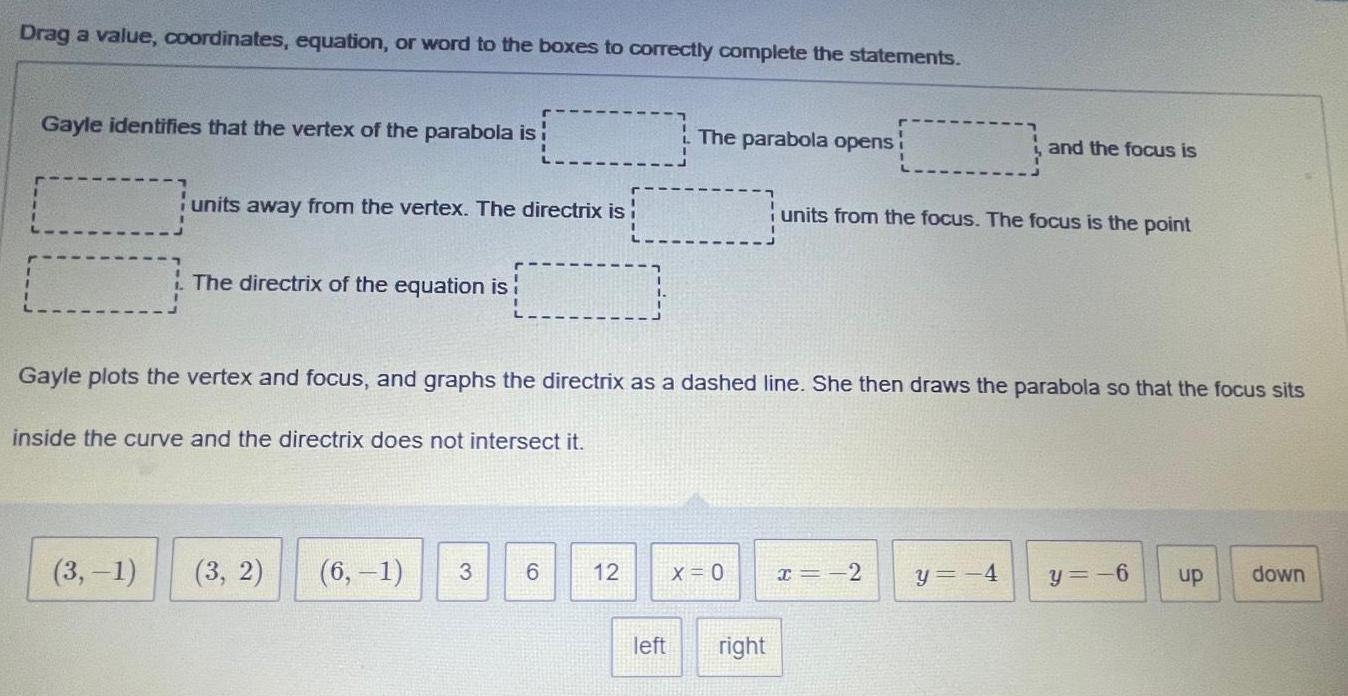Math
Parabola
Drag a value, coordinates, equation, or word to the boxes to correctly complete the statements. Gayle identifies that the vertex of the parabola is! units away from the vertex. The directrix is (3,-1) The directrix of the equation is! (3, 2) Gayle plots the vertex and focus, and graphs the directrix as a dashed line. She then draws the parabola so that the focus sits inside the curve and the directrix does not intersect it.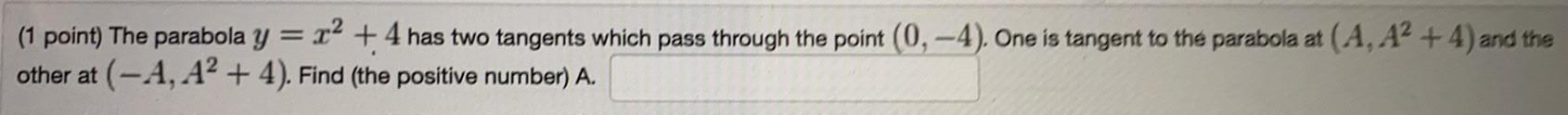Math
Parabola
The parabola y = x² +4 has two tangents which pass through the point (0, -4). One is tangent to the parabola at (A, A²+4) and the other at (-A, A² + 4). Find (the positive number) A.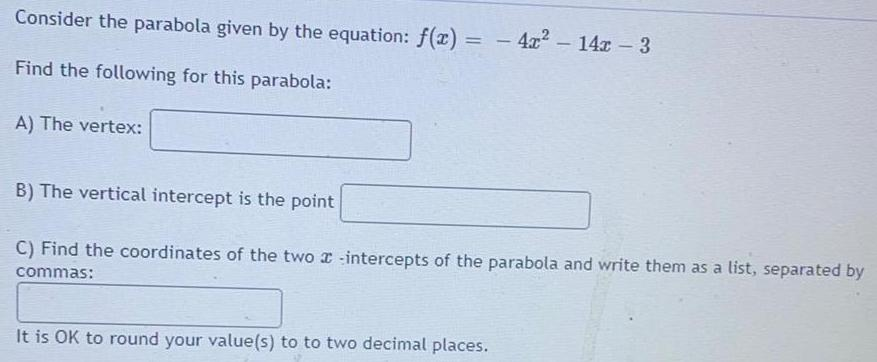Math
Parabola
Consider the parabola given by the equation: f(x) = - 4x² - 14x - 3 Find the following for this parabola: A) The vertex: B) The vertical intercept is the point C) Find the coordinates of the two intercepts of the parabola and write them as a list, separated by commas: It is OK to round your value(s) to to two decimal places.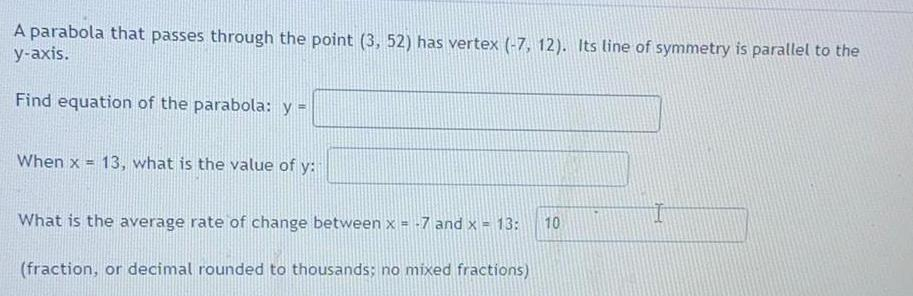Math
Parabola
A parabola that passes through the point (3, 52) has vertex (-7, 12). Its line of symmetry is parallel to the y-axis. Find equation of the parabola: y = When x = 13, what is the value of y: What is the average rate of change between x = -7 and x = 13: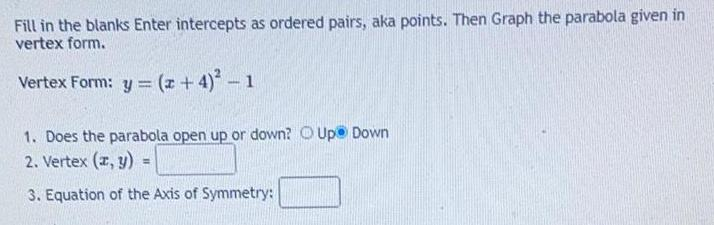Math
Parabola
Fill in the blanks Enter intercepts as ordered pairs, aka points. Then Graph the parabola given in vertex form. Vertex Form: y = (x + 4)² - 1 1. Does the parabola open up or down? Up Down 2. Vertex (x, y) = 3. Equation of the Axis of Symmetry: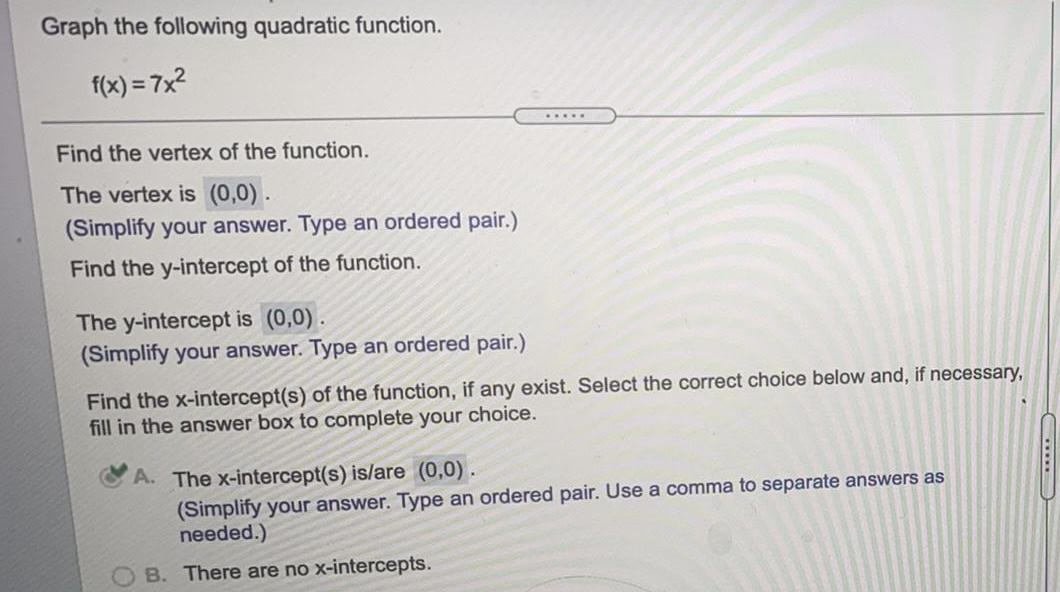Math
Parabola
Graph the following quadratic function. f(x) = 7x² Find the vertex of the function. The vertex is (0,0). (Simplify your answer. Type an ordered pair.) Find the y-intercept of the function. The y-intercept is (0,0). (Simplify your answer. Type an ordered pair.) Find the x-intercept(s) of the function, if any exist. Select the correct choice below and, if necessary, fill in the answer box to complete your choice. A. The x-intercept(s) is/are (0,0). (Simplify your answer. Type an ordered pair. Use a comma to separate answers as needed.) B. There are no x-intercepts.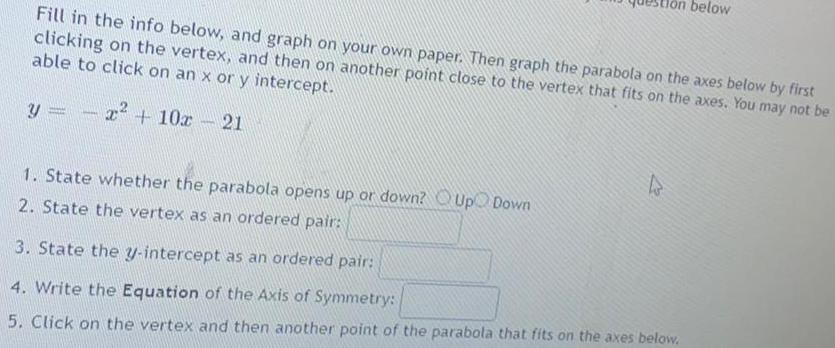Math
Parabola
Fill in the info below, and graph on your own paper. Then graph the parabola on the axes below by first clicking on the vertex, and then on another point close to the vertex that fits on the axes. You may not be able to click on an x or y intercept. y = -x² + 10x - 21 1. State whether the parabola opens up or down? 2. State the vertex as an ordered pair: 3. State the y-intercept as an ordered pair: 4. Write the Equation of the Axis of Symmetry: 5. Click on the vertex and then another point of the parabola that fits on the axes below.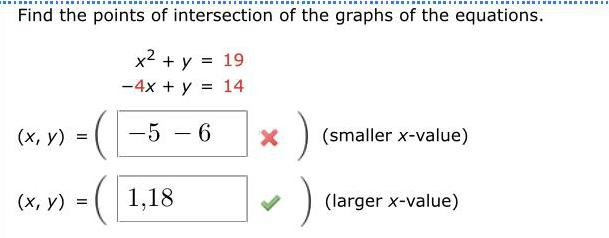Math
Parabola
Find the points of intersection of the graphs of the equations. x² + y = 19 -4x + y = 14 (x, y)= (smaller x-value) (x, y) = (larger x-value)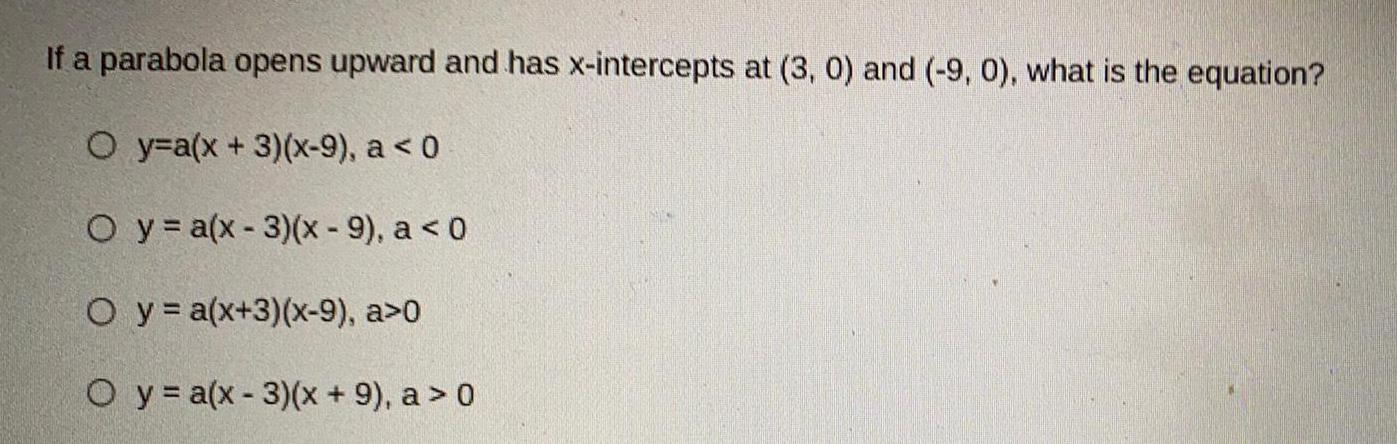Math
Parabola
If a parabola opens upward and has x-intercepts at (3, 0) and (-9, 0), what is the equation? y=a(x+3)(x-9), a < 0 y = a(x-3)(x - 9), a < 0 y = a(x+3)(x-9), a>0 y = a(x-3)(x + 9), a > 0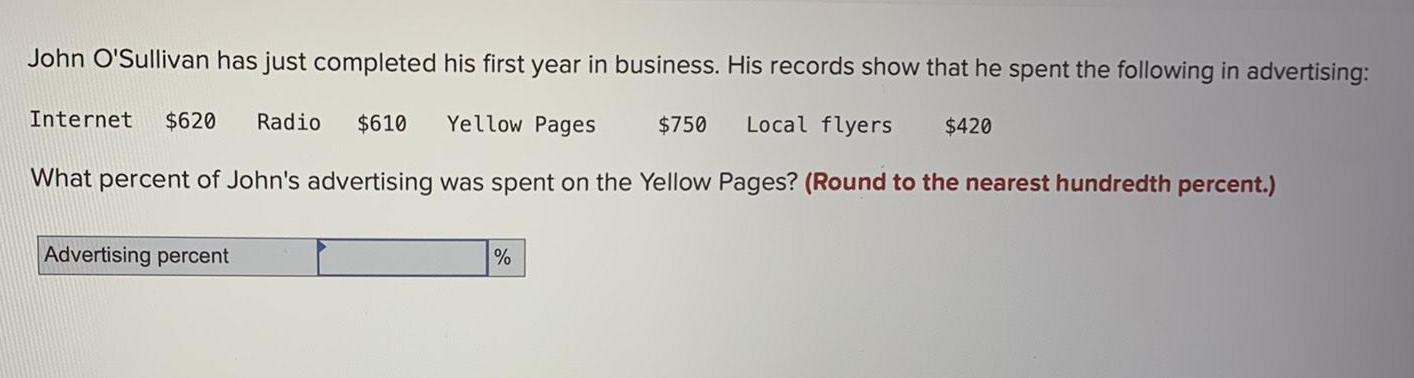Math
Parabola
John O'Sullivan has just completed his first year in business. His records show that he spent the following in advertising: Internet \$620 Radio \$610 Yellow Pages \$750 Local flyers \$420 What percent of John's advertising was spent on the Yellow Pages? (Round to the nearest hundredth percent.)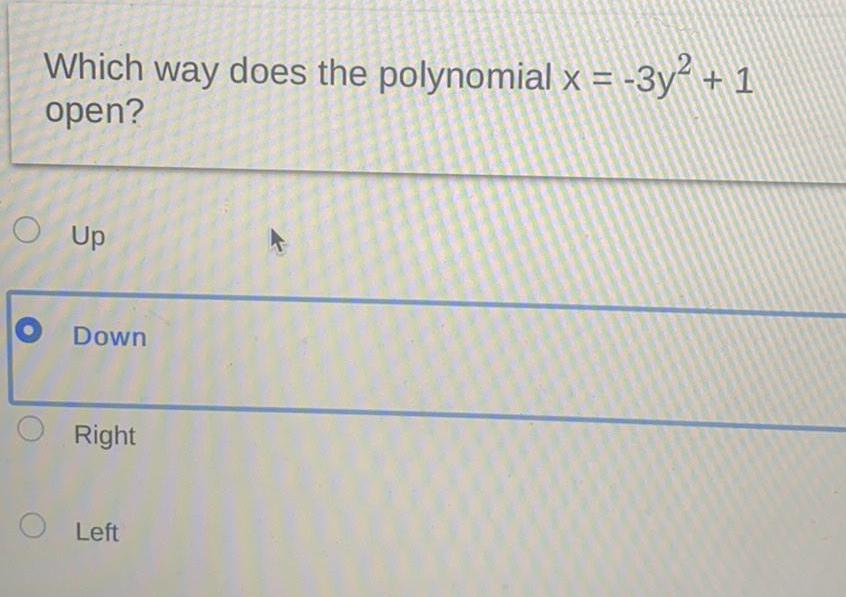Math
Parabola
Which way does the polynomial x = -3y² + 1 open? Up Down Right Left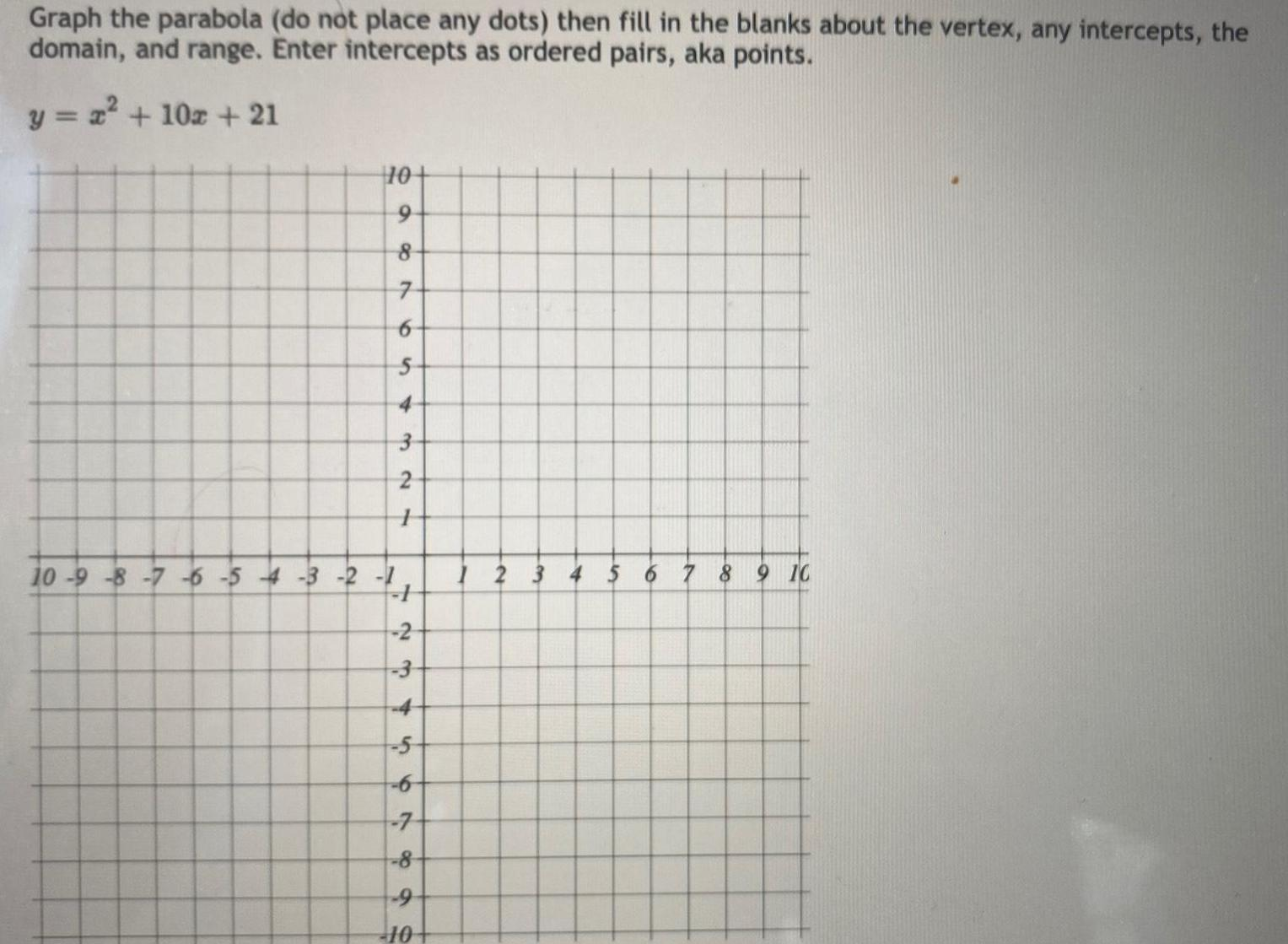Math
Parabola
Graph the parabola (do not place any dots) then fill in the blanks about the vertex, any intercepts, the domain, and range. Enter intercepts as ordered pairs, aka points. y = x² + 10x + 21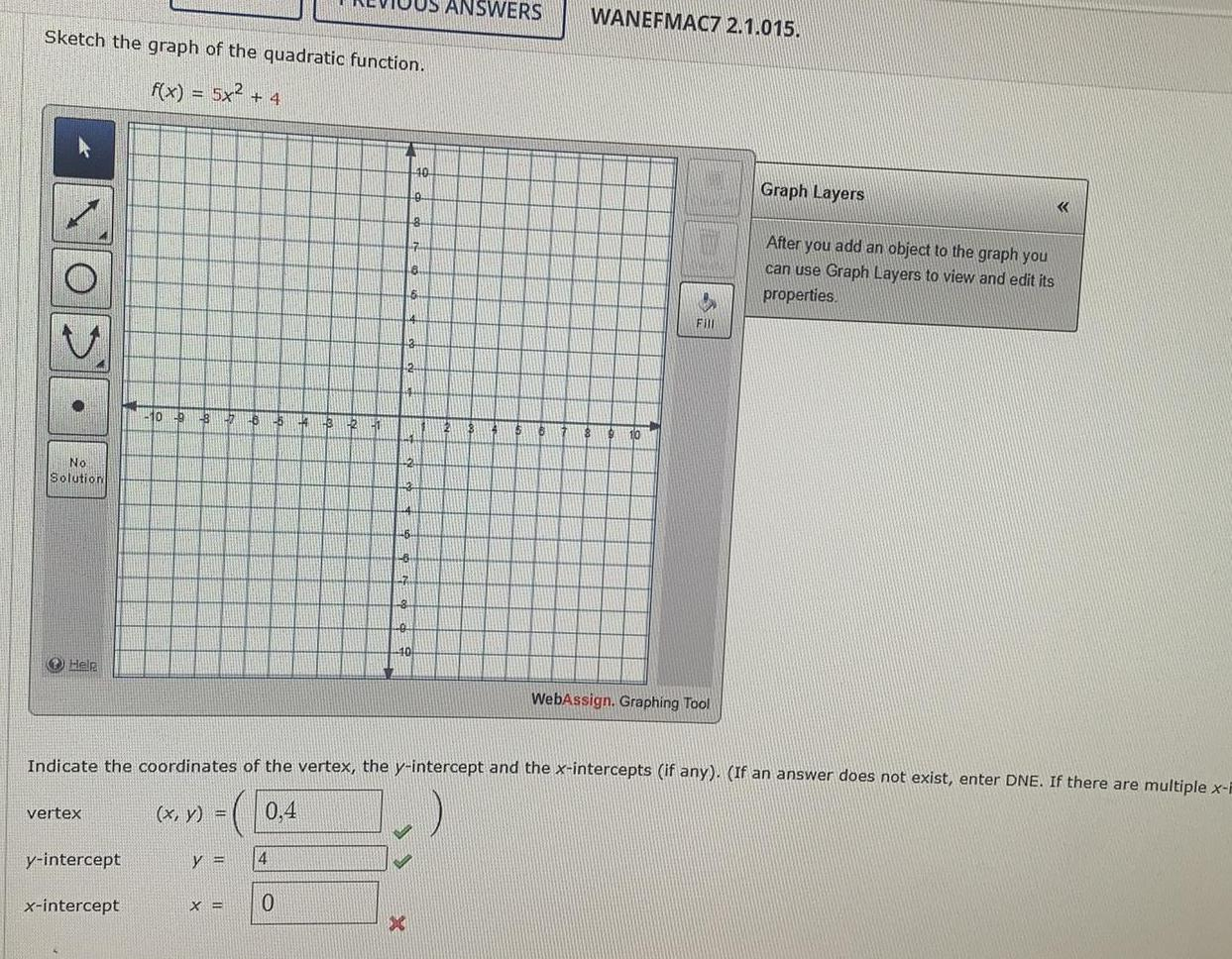Math
Parabola
Sketch the graph of the quadratic function. f(x) = 5x² + 4 Indicate the coordinates of the vertex, the y-intercept and the x-intercepts (if any). (If an answer does not exist, enter DNE. If there are multiple x-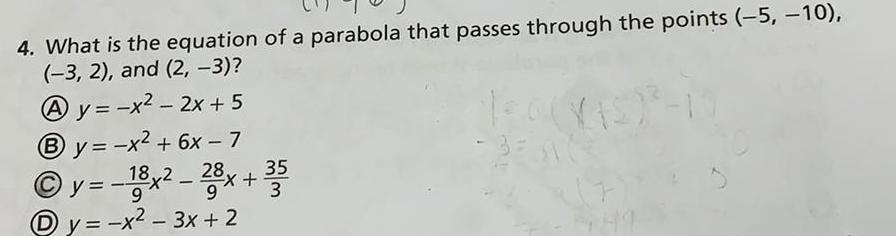Math
Parabola
4. What is the equation of a parabola that passes through the points (-5, -10), (-3, 2), and (2, -3)? Ay=-x² - 2x + 5 By=-x² + 6x - 7 Ⓒy=-18x²-28x +35 9 y = -x² - 3x + 2 (7) 5,49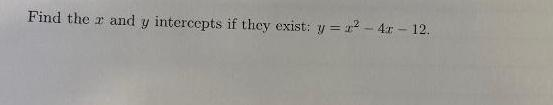Math
Parabola
Find the r and y intercepts if they exist: y = x²-4x - 12.